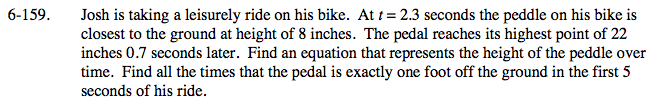### Home > PC > Chapter 6 > Lesson 6.4.2 > Problem6-159

6-159.

Josh is taking a leisurely ride on his bike. At t = 2.3 seconds the peddle on his bike is closest to the ground at height of 8 inches. The pedal reaches its highest point of 22 inches 0.7 seconds later. Find an equation that represents the height of the peddle over time. Find all the times that the pedal is exactly one foot off the ground in the first 5 seconds of his ride. Homework Help ✎Draw a sketch of the situation. From the sketch, find:
1. Amplitude
2. Vertical Shift
3. Horizontal Shift
4. Period
5. From the period, find the frequency (b) which is needed for the equation.
6. Use the general form for the sine or cosine to write the equation.
7. Set your function equal to 12 inches and solve.